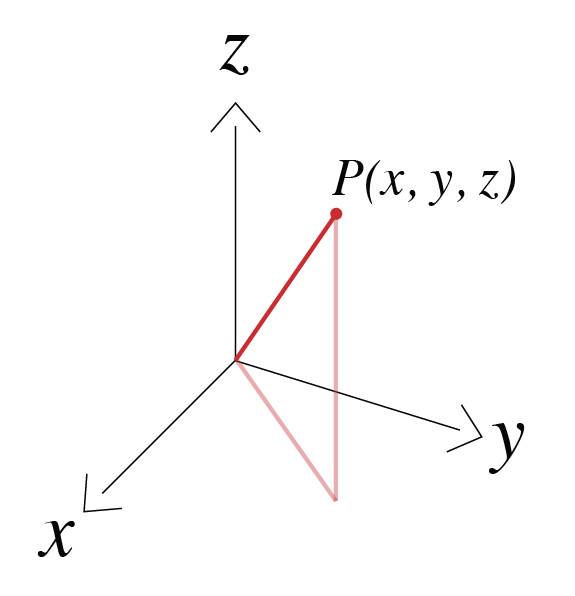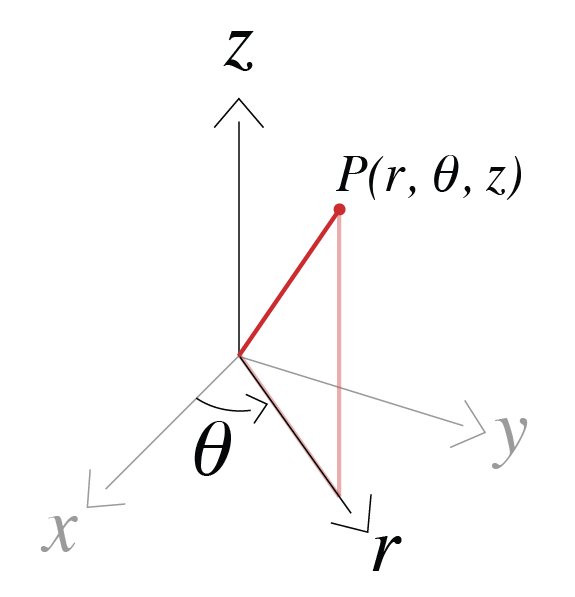# Conventions#

## Coordinate systems#

### Cartesian#

We use a right-handed coordinate system $$(x, y, z)$$ with z-positive up as shown in the Figure below.### Cylindrical#

We again work with z-positive up and use $$\theta$$ to denote the azimuthal angle, thus the coordinate system is defined as $$(r, \theta, z)$$.### Spherical#

We use $$r$$ for the radial direction, $$\theta$$ for the azimuthal direction, and $$\phi$$ for the polar direction as shown in the figure below.## Fourier Transform#

For analysis and solutions in the frequency domain we use the $$e^{i \omega t}$$ Fourier transform convention. Thus, we define our Fourier Transform pair as

\begin{align}\begin{aligned}\begin{split}F(\omega) = \int_{-\infty}^{\infty} f(t) e^{- i \omega t} dt \\\end{split}\\f(t) = \frac{1}{2\pi}\int_{-\infty}^{\infty} F(\omega) e^{i \omega t} d \omega\end{aligned}\end{align}

where $$\omega$$ is angular frequency, $$t$$ is time, $$F(\omega)$$ is the function defined in the frequency domain and $$f(t)$$ is the function defined in the time domain.